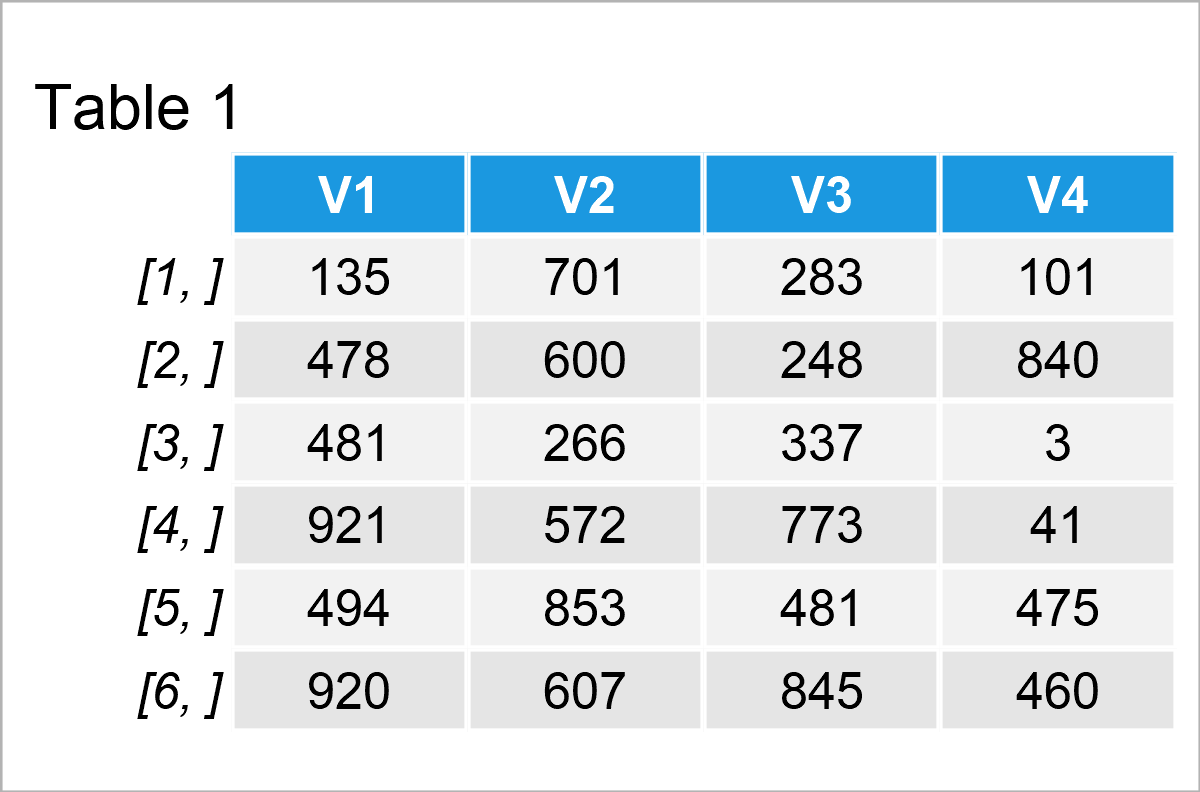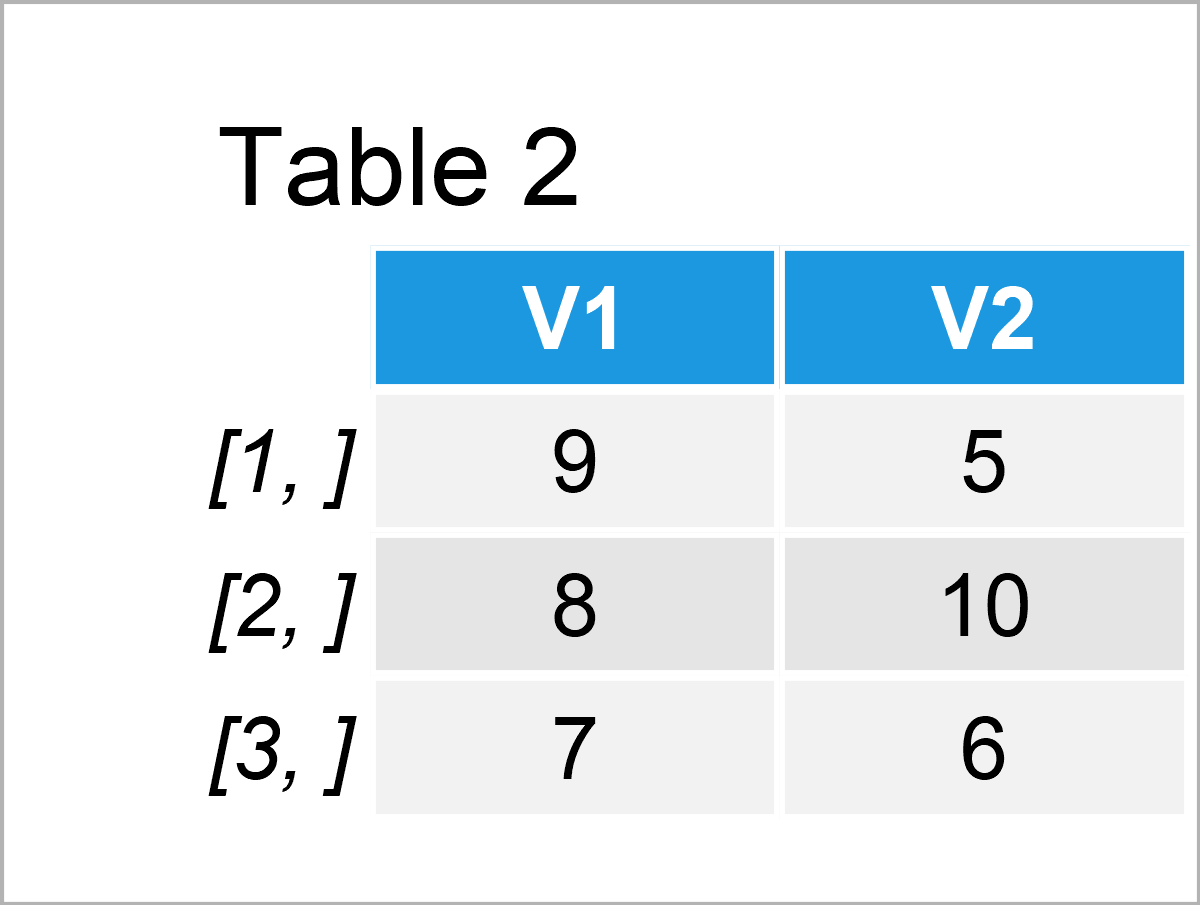# Introduction to the random Package in R (3 Examples)

On this page I’ll explain how to draw random numbers and character strings using the random package in the R programming language.

The article will consist of these contents:

Let’s do this!

## Basic Information about the random Package

The random package, created by Dirk Eddelbuettel, allows the user to draw true random numbers by sampling from atmospheric noise via radio tuned to an unused broadcasting frequency combined with a skew correction algorithm based on the work of John von Neumann (a service provided by the website random.org).

In contrast to pseudo-random numbers, real random numbers cannot be decrypted with a random seed, which might be preferable in terms of security and hacker-protection. Furthermore, true random values are closer to nature and might therefore be advantageous for random experiments and simulation studies.

Before we dive into the exemplifying R codes…

• Here you can find the documentation of the random package.
• Here you can find the CRAN page of the random package.

Make sure to check out the previous links for more instructions on how to use the random package in the R programming language.

In order to use the functions of the random package, we have to install and load the package first:

```install.packages("random")                # Install & load random package
library("random")```

So far so good, let’s jump into the example codes in R!

## Example 1: Create Data Set Containing Random Integers with Duplicates

In the first example, I’ll explain how to construct a random data set that contains random integers.

For this task, we can use the randomNumbers function provided by the random package. Within this function, we can specify several arguments such as the sample size, the minimum value, tha maximum value, and the number of columns.

Have a look at the example code below:

```data1 <- randomNumbers(n = 200,            # Apply randomNumbers() Function
min = 0,
max = 1000,
col = 4)Table 1 illustrates the first six rows of our random data, i.e. the RStudio console output of the previous R code. As you can see, we have created a data set containing four columns and 200 rows.

Note that the integer values in our data could be duplicated. In the next example, I’ll show how to create a data set where each value appears only once.

## Example 2: Create Data Set Containing Random Sequence without Duplicates

Example 2 explains how to create a data matrix with unique random values.

For this, we can apply the randomSequence function of the random package. Within the function, we can specify the minimum and maximum values as well as the number of columns.

```data2 <- randomSequence(min = 5,           # Apply randomSequence() Function
max = 10,
col = 2)As you can see based on Table 2, we have created a data set consisting of three rows and two variables. Each value appears only once.

## Example 3: Create Vector of Random Character Strings with Certain Length

The random package can also be used to sample random character strings.

To do this, we have to use the randomStrings function. Note that we are also using the as.vector function to convert the output to a vector object instead of a matrix:

```vec1 <- as.vector(randomStrings(n = 7,     # Apply randomStrings() Function
len = 3))
vec1                                       # Print vector of random strings
#  "W8p" "S9v" "H4u" "nh0" "JXz" "VIm" "8N9"```

The previous R code has simulated a character string vector containing seven vector elements. Each of these elements contains a random character string with a length of three.

## Video & Further Resources

Do you want to learn more about the random package? Then I recommend watching the following video of my YouTube channel. In the video, I explain the examples of this article in some more detail.

In addition, you may have a look at the other posts on Statistics Globe. I have released several tutorials on the simulation of random numbers already:

On this page you have learned how to draw true random values using the random package in R.

Subscribe to the Statistics Globe Newsletter

•A.h.khan
August 21, 2021 12:50 pm

How to avoid duplicates in random numbers

•August 23, 2021 6:53 am

Hey,

As far as I know, the randomNumbers function does not provide an option for random number generation without duplicates.

You may generate pseudo-random numbers with an R code as shown below:

`sample(1:1000, 10, replace = FALSE)`

Regards

Joachim